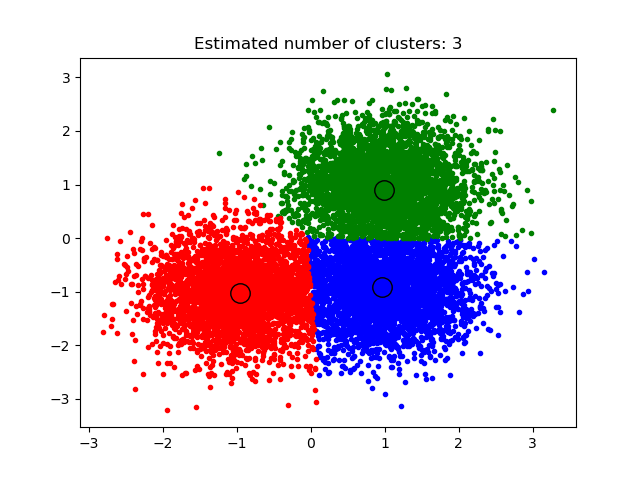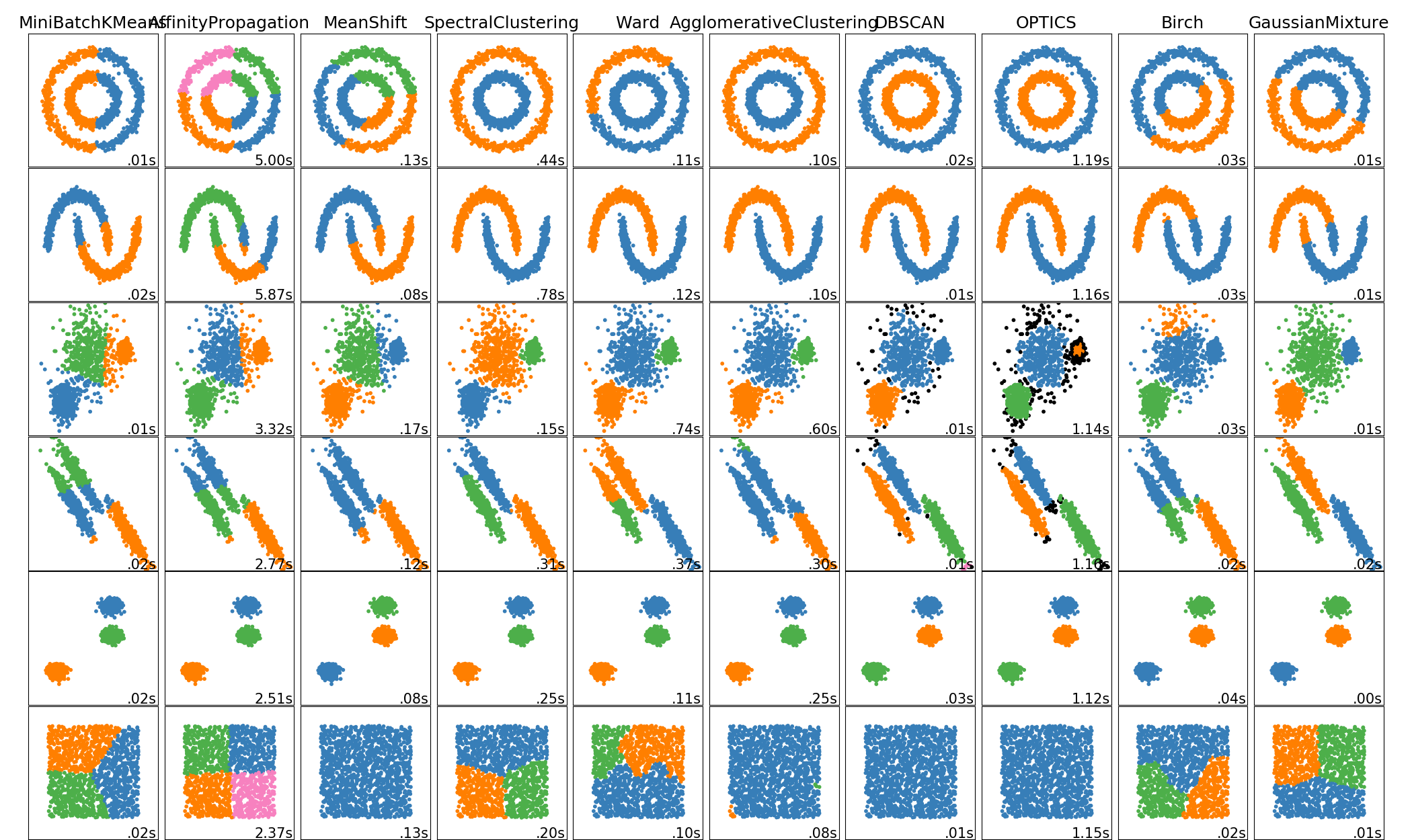# sklearn.cluster.MeanShift¶

class sklearn.cluster.MeanShift(*, bandwidth=None, seeds=None, bin_seeding=False, min_bin_freq=1, cluster_all=True, n_jobs=None, max_iter=300)

[源码]

bandwidth float, default=None
RBF核中使用的带宽。如果不给出带宽，则使用sklearn.cluster.estimate_bandwidth来估算带宽；有关可伸缩性的提示，请参阅该函数的文档(另请参阅下面的Notes)。
seeds array-like of shape (n_samples, n_features), default=None

bin_seeding bool, default=False

min_bin_freq int, default=1

cluster_all bool, default=True

n_jobs int, default=None

None意味着1，除非在joblib.parallel_backend上下文中。-1指使用所有处理器。有关详细信息，请参阅Glossary
max_iter int, default=300

cluster_centers_ array, [n_clusters, n_features]

labels_ array of shape (n_samples,)

n_iter_ int

Dorin Comaniciu and Peter Meer, “Mean Shift: A robust approach toward feature space analysis”. IEEE Transactions on Pattern Analysis and Machine Intelligence. 2002. pp. 603-619.

>>> from sklearn.cluster import MeanShift>>> import numpy as np>>> X = np.array([[1, 1], [2, 1], [1, 0],...               [4, 7], [3, 5], [3, 6]])>>> clustering = MeanShift(bandwidth=2).fit(X)>>> clustering.labels_array([1, 1, 1, 0, 0, 0])>>> clustering.predict([[0, 0], [5, 5]])array([1, 0])>>> clusteringMeanShift(bandwidth=2)

fit(self, X[, y]) 执行聚类
fit_predict(self, X[, y]) 在X上执行聚类并返回聚类标签
get_params(self[, deep]) 获取此估计器的参数
predict(self, X) 预测X中每个样本所属的最接近的聚类
set_params(self, **params) 设置此估计器的参数
__init__(self, *, bandwidth=None, seeds=None, bin_seeding=False, min_bin_freq=1, cluster_all=True, n_jobs=None, max_iter=300)

[源码]

fit(self, X, y=None)

[源码]

X array-like of shape (n_samples, n_features)

y Ignored
fit_predict(self, X, y=None)

[源码]

X array-like of shape (n_samples, n_features)

y Ignored

labels ndarray of shape (n_samples,)

get_params(self, deep=True)

[源码]

deep bool, default=True

params mapping of string to any

predict(self, X)

[源码]

X {array-like, sparse matrix}, shape=[n_samples, n_features]

labels array, shape [n_samples,]

set_params(self, **params)

[源码]

**params dict

self object

## sklearn.cluster.MeanShift使用示例¶